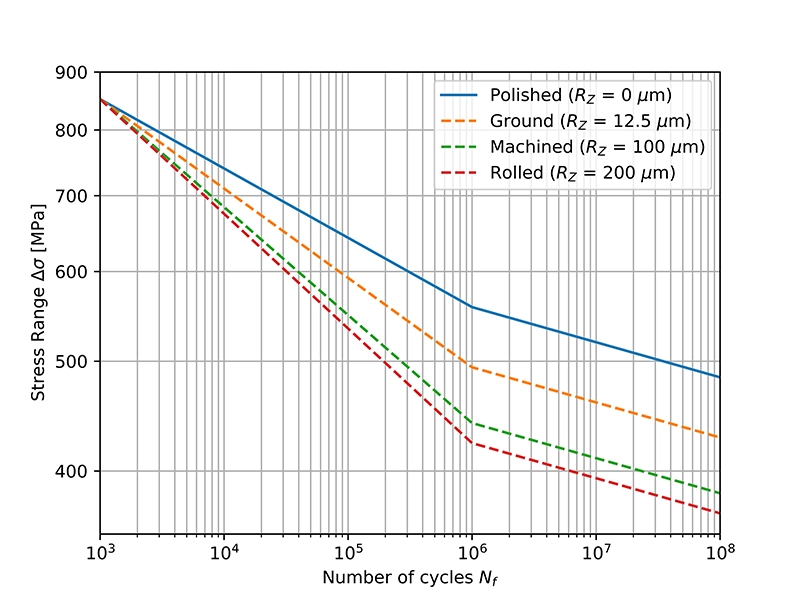# Surface Roughness Factor KR

Fatigue tests of specimens are usually done on components with mirror polished surfaces. However, a component with a larger surface roughness will have a reduced fatigue strength. To account for the effect of this surface roughness to the fatigue strength of a material, a roughness factor KR is used. This surface roughness factor is used to adjust the material S-N curve. This article describes the FKM method for obtaining the surface roughness factor and how to apply this factor to the S-N curve.

## FKM surface roughness factor

The FKM-guideline Analytical Strength Assessment defines the roughness factor KR as follows:

$$K_R=1-a_R \cdot log_{10}(R_Z) \cdot log_{10}\left(\frac {2R_m}{R_{m,N,min}}\right)$$

With:

• RZ the surface roughness in µm according to DIN 4768 (see Table 2)
• Rm the tensile strength in MPa
• aR a constant (see Table 1)
• Rm,N,min the minimum tensile strength in MPa (see Table 1)

The roughness factor KR = 1 for a polished surface. Surface roughnesses higher than that of a polished surface will have values for KR < 1.

Table 1 below gives the values for Rm,N,min and for constant aR for different material types.

Table 1. Constant aR and minimum tensile strength Rm,N,min for different material types (according to FKM)
GS = Cast steel and heat treatable cast steel, for general purposes
GGG = Nodular cast iron
GT = Malleable cast iron
GG = Cast iron with lamellar graphite (gray cast iron)
Material Type aR Rm,N,min [MPa]
Steel 0.22 400
GS 0.20 400
GGG 0.16 400
GT 0.12 350
GG 0.06 100
Wrought aluminium alloys 0.22 133
Cast aluminium alloys 0.20 133

Table 2 below provides some indicative values for the surface roughness RZ of different surface finishes.

Table 2. Indicative values for different surface finishes (according to DIN 4768)
Surface Condition Rz [µm]
Polished 0
Ground 12.5
Machined 100
Poor machined 200
Rolled 200
Cast 200

## Example calculation

As an example, let's look at a steel with a tensile strength Rm = 600 MPa and with a machined surface.

In Table 1 we find for Steel: aR = 0.22 and Rm,N,min = 400 MPa. Table 2 gives us RZ = 100 µm for a machined surface.

Putting these values in the above equation gives us:

$$K_R=1-0.22 \cdot log_{10}(100)\cdot log_{10}\left( \frac{2 \cdot 600}{400}\right)$$

and we get KR = 0.79 as result.

## Construction of the S-N curve

Before we get to how the S-N curve is adjusted for the surface roughness factor, let's look at how the S-N curve is constructed (see Figure 1 below - click for larger image).

For the number of cycles Nf between 103 and Nc1 cycles, the S-N curve is defined as:

$$\Delta \sigma(N_f) = SRI1 \cdot N_f^{b_1}$$

The stress range Δσ is a function of the number of cycles Nf. SRI1 is the stress range intercept at 1 cycle and b1 is the slope, which is negative. The parameter Nc1 is called the Fatigue Transition Point and is basically the number of cycles by which a kink in the fatigue curve is noticeable. The value of Nc1 is usually around 106 - 107 cycles.

For Nf > Nc1, the S-N curve is defined as:

$$\Delta \sigma(N_f) = SRI2 \cdot N_f^{b_2}$$

SRI2 is the stress range intercept at 1 cycle and b2 is the slope, which is negative, for the second part of the S-N curve.

The parameters SRI1, Nc1, b1 and b2 are material parameters derived from the fatigue test data. SRI2 can be derived as follows:

$$SRI2 = SRI1 \cdot (N_{c1})^{b_1-b_2}$$

## S-N curve adjusted for the surface roughness factor

The surface condition has the greatest effect in the high-cycle regime and becomes progressively smaller towards the low-cycle regime. S-N curves are usually adjusted for the surface roughness by changing the slope b1 in the first part of the S-N curve (see figure below) and keeping the fatigue strength at 1000 cycles the same. The slope b2 of the second part of the S-N curve remains unchanged.Figure 2. S-N curve of a polished steel specimen, plotted against the corrected S-N curves for different surface finishes. b1 = -0.0612 | b2 = -0.0310 | SRI1 = 1300 MPa | UTS = 600 MPa | Nc1 = 106 cycles

So, let's find out how the equation of the S-N curve in Figure 2 is modified. For the unadjusted curve (polished surface), the stress range at Nc1 cycles is:

$$\Delta \sigma(N_{c1}) = 1300 \cdot (10^6)^{-0.0612}=558.14 \text{ MPa}$$

The stress range Δσ' at Nc1 cycles for a machined part becomes in our case (with KR = 0.79, as calculated in the beginning):

$$\Delta \sigma^{'}(N_{c1}) = K_R \cdot 558.14 = 440.97 \text{ MPa}$$

SRI1' and b1' for the first part of the modified curve can be determined from the two equalities below:

$$SRI1 \cdot (10^3)^{b_1} = 851.81 = SRI1^{'} \cdot (10^3)^{b^{'}_1}$$

$$\Delta \sigma^{'}(N_{c1}) = 440.97 = SRI1^{'} \cdot (10^6)^{b_1^{'}}$$

When we solve for SRI1' and b1', we find:

SRI1' = 1645 MPa and b1' = -0.0953

Since b2' = b2, we can solve below equation for SRI2':

$$\Delta \sigma^{'}(N_{c1}) = 440.97 = SRI2^{'} \cdot (10^6)^{b_2}$$

which gives us SRI2' = 677 MPa.

The equation of the modified S-N curve between 103 and Nc1 cycles is then:

$$\Delta \sigma(N_{f}) = 1645 \cdot (N_f)^{-0.0953}$$

And in the region for Nf > Nc1:

$$\Delta \sigma(N_{f}) = 677 \cdot (N_f)^{-0.0310}$$